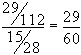LEGACY CONTENT. If you are looking for Voteview.com, PLEASE CLICK HERE

This site is an archived version of Voteview.com archived from University of Georgia on May 23, 2017. This point-in-time capture includes all files publicly linked on Voteview.com at that time. We provide access to this content as a service to ensure that past users of Voteview.com have access to historical files. This content will remain online until at least January 1st, 2018. UCLA provides no warranty or guarantee of access to these files.

Probability and Statistics                      Name__________________________
Spring 1997 Flex-Mode 45-733
Practice Midterm
Keith Poole

(10 Points)

1. Suppose we have a discrete bivariate probability distribution

f(x,y) =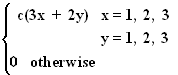a. Find c.
```
y
1   2   3
------------
1 | 5   7   9 |21
|           |
x  2 | 8  10  12 |30
|           |
3 |11  13  15 |39
|           |
---------------
24  30  36 |90
```

Hence, c = 1/90

b. Find f1(x) and f2(y).

f1(x) =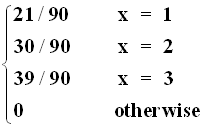f2(x) =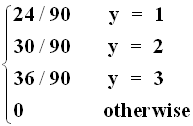c. Are X, Y independent?

No. f(1,1) =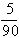¹ f1(1) * f2(1) =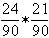Probability and Statistics                      Name__________________________
Spring 1997 Flex-Mode 45-733
Practice Midterm
Keith Poole

(10 Points)

2. Suppose in a certain city that 40% of the eligible voters are Republicans, 40% are Democrats, and 20% are Reform party members. In the last election 85% of the Republicans voted, 75% of the Democrats voted, and 40% of the Reform party members voted. A person is chosen at random from this population and we learn that she voted in the last election. What is the probability that she is a Republican?

A1 = Republican
A2 = Democrat
A3 = Reform
V = Voted

P(A1) = 0.4       P(V|A1) = 0.85
P(A2) = 0.4       P(V|A2) = 0.75
P(A3) = 0.2       P(V|A3) = 0.4

P(A1|V) =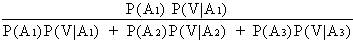=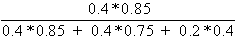Probability and Statistics                      Name__________________________
Spring 1997 Flex-Mode 45-733
Practice Midterm
Keith Poole

(10 Points)

3. Suppose we have the continuous probability distribution

f(x) =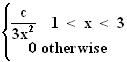a. Find c.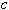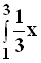-2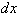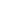= -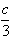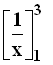= -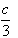[ 1/3 - 1] =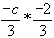=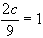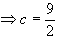b. Find VAR(X).

E[X] =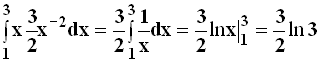E[X2] =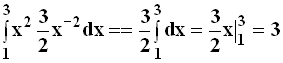Var[X] = 3 - [3/2 ln(3)]2

Probability and Statistics                      Name__________________________
Spring 1997 Flex-Mode 45-733
Practice Midterm
Keith Poole

(10 Points)

4. Your company is very concerned about a case of alleged job discrimination by one of the company�s supervisors. It is alleged that in assigning 30 workers to five different jobs, the supervisor assigned workers in a discriminatory manner. The five jobs require 7 workers, 8 workers, 6 workers, 3 workers, and 6 workers respectively. The job requiring 8 workers is considered to be abominable employment. In your investigation you find that the supervisor has assigned all the minority workers, 4 are from one minority group and 4 are from a second minority group, to the second job (the one requiring 8 workers). The supervisor claims that he assigned the jobs randomly. What is the probability he is telling the truth?

This is a lot like Exercise 2.10 and HW#2.42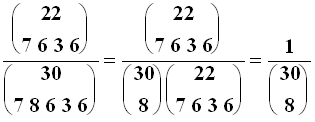To see this, note that there are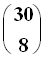ways of choosing 8 workers for Job II and only one of which has all 8 minority employees being chosen.

Probability and Statistics                      Name__________________________
Spring 1997 Flex-Mode 45-733
Practice Midterm
Keith Poole

(10 Points)

5. Approximately 10% of computer chips coming off an assembly line have serious flaws. If four chips are randomly selected, find the probability distribution for the number of defective chips in the sample and calculate the mean of this distribution.

This is a binomial with n = 4 and p = 0.1.

Let Z = number of defective chips. Therefore,

f(z) =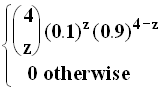z = 0, 1, 2, 3, 4

Hence E(Z) = np = .4.

Probability and Statistics                      Name__________________________
Spring 1997 Flex-Mode 45-733
Practice Midterm
Keith Poole

(10 Points)

6. Consider the following portion of an electric circuit with seven relays. Current will flow from point A to point B if there is at least one complete path when the relays are activated. The relays are turned on simultaneously and their failures are independent of one another. The relays are identical in every respect and the probability that a relay fails is 0.2. What is the probability that current will flow from A to B when the relays are activated?

```
|--2---3--|
|--1--|         |---|
|     |----4----|   |
|                   |
A---|-------5------6----|---B
|                   |
|----------7--------|
```

There are three paths: upper, middle, lower (U, M, C). For the circuit to work:

P[Works] = 1 - P[All three paths fail]

P[U works] = 0.8*[0.64 + 0.8 - 0.8*0.64] = 0.7424
P[M works] = 0.64
P[L works] = 0.8
P[Works] = 1 - (0.2576*0.36*0.2) = 1 - 0.01855 = 0.9815

Probability and Statistics                      Name__________________________
Spring 1997 Flex-Mode 45-733
Practice Midterm
Keith Poole

(10 Points)

7. You roll 10 dice. What is the probability that exactly two of one number, three of a second number, and five of a third number appear (e.g., 3322255555, 4411133333, etc.).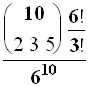Probability and Statistics                      Name__________________________
Spring 1997 Flex-Mode 45-733
Practice Midterm
Keith Poole

(10 Points)

8. A new test for heart disease is 99% accurate in that if the person has the disease the test will detect it with probability .99. If the person does not have the disease the test will report that the person has the disease with probability .4 (this is known as a "false positive"). The proportion of the population that has heart disease is .10. If a person is chosen randomly from the population and the test indicates that he does not have the disease, what is the probability that he in fact does have the disease?

Let A = "positive result" and D = "Disease"

P(A|D) = 0.99       P(Ac|D) = 0.01
P(A|Dc) = 0.4       P(Ac|Dc) = 0.6
P(D) = 0.1       P(Dc) = 0.9

Hence, P(D|Ac) =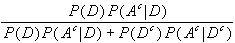=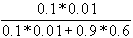Probability and Statistics                      Name__________________________
Spring 1997 Flex-Mode 45-733
Practice Midterm
Keith Poole

(5 Points)

9. Supposed we have the probability distribution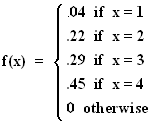Find F(x).

F(x) =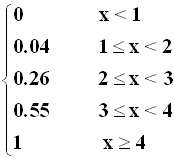Probability and Statistics                      Name__________________________
Spring 1997 Flex-Mode 45-733
Practice Midterm
Keith Poole

(10 Points)

10. Suppose we have the bivariate continuous probability function

f(x,y) =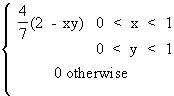Find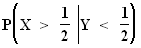P[X > 1/2|Y < 1/2] =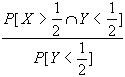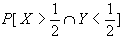=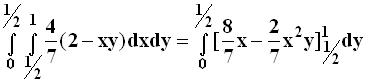=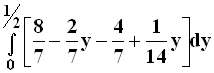=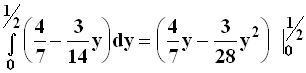=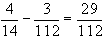P[Y<1/2] =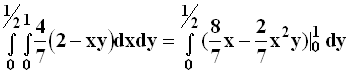=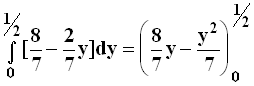=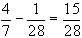Therefore,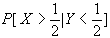=